# G2 (mathematics)

In mathematics, G2 is the name of three simple Lie groups (a complex form, a compact real form and a split real form), their Lie algebras, as well as some algebraic groups. They are the smallest of the five exceptional simple Lie groups. G2 has rank 2 and dimension 14. It has two fundamental representations, with dimension 7 and 14.

The compact form of G2 can be described as the automorphism group of the octonion algebra or, equivalently, as the subgroup of SO(7) that preserves any chosen particular vector in its 8-dimensional real spinor representation.

## History

The Lie algebra, being the smallest exceptional simple Lie algebra, was the first of these to be discovered in the attempt to classify simple Lie algebras. On May 23, 1887, Wilhelm Killing wrote a letter to Friedrich Engel saying that he had found a 14-dimensional simple Lie algebra, which we now call.

In 1893, Élie Cartan published a note describing an open set inequipped with a 2-dimensional distribution—that is, a smoothly varying field of 2-dimensional subspaces of the tangent space—for which the Lie algebraappears as the infinitesimal symmetries. In the same year, in the same journal, Engel noticed the same thing. Later it was discovered that the 2-dimensional distribution is closely related to a ball rolling on another ball. The space of configurations of the rolling ball is 5-dimensional, with a 2-dimensional distribution that describes motions of the ball where it rolls without slipping or twisting.

In 1900, Engel discovered that a generic antisymmetric trilinear form (or 3-form) on a 7-dimensional complex vector space is preserved by a group isomorphic to the complex form of G2.

In 1908 Cartan mentioned that the automorphism group of the octonions is a 14-dimensional simple Lie group. In 1914 he stated that this is the compact real form of G2.

In older books and papers, G2 is sometimes denoted by E2.

## Real forms

There are 3 simple real Lie algebras associated with this root system:

• The underlying real Lie algebra of the complex Lie algebra G2 has dimension 28. It has complex conjugation as an outer automorphism and is simply connected. The maximal compact subgroup of its associated group is the compact form of G2.
• The Lie algebra of the compact form is 14-dimensional. The associated Lie group has no outer automorphisms, no center, and is simply connected and compact.
• The Lie algebra of the non-compact (split) form has dimension 14. The associated simple Lie group has fundamental group of order 2 and its outer automorphism group is the trivial group. Its maximal compact subgroup is SU(2) × SU(2)/(−1,−1). It has a non-algebraic double cover that is simply connected.

## Algebra

### Dynkin diagram and Cartan matrix

The Dynkin diagram for G2 is given by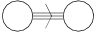.

Its Cartan matrix is:### Roots of G2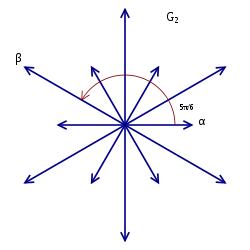The 12 vector root system of G2 in 2 dimensions.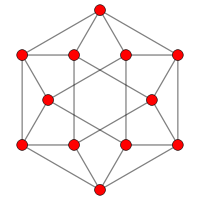The A2 Coxeter plane projection of the 12 vertices of the cuboctahedron contain the same 2D vector arrangement.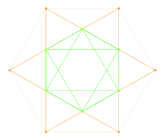Graph of G2 as a subgroup of F4 and E8 projected into the Coxeter plane

Although they span a 2-dimensional space, as drawn, it is much more symmetric to consider them as vectors in a 2-dimensional subspace of a three-dimensional space.

 (1,−1,0), (−1,1,0) (1,0,−1), (−1,0,1) (0,1,−1), (0,−1,1) (2,−1,−1), (−2,1,1) (1,−2,1), (−1,2,−1) (1,1,−2), (−1,−1,2)

One set of simple roots, for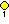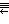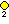is:

(0,1,1), (1,2,1)

### Weyl/Coxeter group

Its Weyl/Coxeter groupis the dihedral group,of order 12. It has minimal faithful degree.

### Special holonomy

G2 is one of the possible special groups that can appear as the holonomy group of a Riemannian metric. The manifolds of G2 holonomy are also called G2-manifolds.

## Polynomial Invariant

G2 is the automorphism group of the following two polynomials in 7 non-commutative variables.(± permutations)

which comes from the octonion algebra. The variables must be non-commutative otherwise the second polynomial would be identically zero.

## Generators

Adding a representation of the 14 generators with coefficients A..N gives the matrix:It's exactly the Lie algebra of the group## Representations

The characters of finite-dimensional representations of the real and complex Lie algebras and Lie groups are all given by the Weyl character formula. The dimensions of the smallest irreducible representations are (sequence A104599 in the OEIS):

1, 7, 14, 27, 64, 77 (twice), 182, 189, 273, 286, 378, 448, 714, 729, 748, 896, 924, 1254, 1547, 1728, 1729, 2079 (twice), 2261, 2926, 3003, 3289, 3542, 4096, 4914, 4928 (twice), 5005, 5103, 6630, 7293, 7371, 7722, 8372, 9177, 9660, 10206, 10556, 11571, 11648, 12096, 13090.

The 14-dimensional representation is the adjoint representation, and the 7-dimensional one is action of G2 on the imaginary octonions.

There are two non-isomorphic irreducible representations of dimensions 77, 2079, 4928, 28652, etc. The fundamental representations are those with dimensions 14 and 7 (corresponding to the two nodes in the Dynkin diagram in the order such that the triple arrow points from the first to the second).

Vogan (1994) described the (infinite-dimensional) unitary irreducible representations of the split real form of G2.

## Finite groups

The group G2(q) is the points of the algebraic group G2 over the finite field Fq. These finite groups were first introduced by Leonard Eugene Dickson in Dickson (1901) for odd q and Dickson (1905) for even q. The order of G2(q) is q6(q6 − 1)(q2 − 1). When q ≠ 2, the group is simple, and when q = 2, it has a simple subgroup of index 2 isomorphic to 2A2(32), and is the automorphism group of a maximal order of the octonions. The Janko group J1 was first constructed as a subgroup of G2(11). Ree (1960) introduced twisted Ree groups 2G2(q) of order q3(q3 + 1)(q − 1) for q = 32n+1, an odd power of 3.

4. John Baez and John Huerta (2014). "G2 and the rolling ball". Trans. Amer. Math. Soc. 366: 5257–5293. arXiv:1205.2447. doi:10.1090/s0002-9947-2014-05977-1.